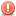##Given an array that contains numbers from 1 to 1+n

Hello I have to do a task, but not sure how to proceed please could someone help me I would be really thankful so the thing I have to do is the following:

You are given an array A of size n that contains numbers in the range
1 to n + 1. We know that each number occurs at most once in A. Because there are only n
numbers in A out of n + 1 possible numbers, exactly one of the numbers is missing.

(a) Give a worst-case O(n) time algorithm that determines the missing number. Also prove
the running time of your algorithm.

(b) If the array A was sorted, we could find the missing number in O(log n) time instead.
Give an algorithm that finds the missing number in a sorted array in O(log n) time and
also prove its running time.